﻿ 通过像素相关性改进的基于均值的密文域可逆信息隐藏 The Mean-Based Ciphertext Domain Reversible Information Hiding Is Improved by Pixel Correlation

Computer Science and Application
Vol. 08  No. 11 ( 2018 ), Article ID: 27422 , 8 pages
10.12677/CSA.2018.811182

The Mean-Based Ciphertext Domain Reversible Information Hiding Is Improved by Pixel Correlation

Shiyi Zhao, Yuan Yuan

Country Graduate School of Tangshan, Southwest Jiaotong University, Tangshan Heibei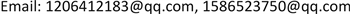Received: Oct. 17th, 2018; accepted: Oct. 30th, 2018; published: Nov. 6th, 2018ABSTRACT

This paper improves the image encryption algorithm and the image embedding capacity. The document divided image into the block columned by 256 * 1. The number of blocks determines the number of bits embedded. The original block is too large, resulting in relatively few embedded data. Therefore, this paper divides the block as small as possible and modifies the embedding method and extracts the information completely according to the neighboring pixels of the average pixel. Using this method, the embedded information increases. Experimental results show that the embedding capacity obtained by this method is almost ten times of that. In this part, we mainly discuss the method of making space after encryption in the algorithm of reversible information hiding based on encryption domain. The proposed scheme is also simple and easy to operate with security and reversibility and privacy protection.

Keywords:Encrypted Image, Image Recovery, Information Hiding, Reversible Data Hiding1. 引言

2. 本文提出的算法

2.1. 计算均值

2.2. 加密图像

$\text{E}\left(\text{i},\text{j}\right)=\left(\text{J}\left(\text{i},\text{j}\right)+\text{R}\left(\text{i},\text{j}\right)\right)\mathrm{mod}\text{256}$ (3)

2.3. 嵌入信息

2.4. 解密图像

$\text{D}\left(\text{i},\text{j}\right)=\left(\text{C}\left(\text{i},\text{j}\right)-\text{R}\left(\text{i},\text{j}\right)\right)\mathrm{mod}\text{256}$ (4)

2.5. 提取信息恢复图像

$\text{first}=\text{round}\left(\text{s}1\text{j}\ast \text{x}-{\sum }_{\text{i}=2}^{\text{x}}\text{sij}\right)$ (5)

$\text{f}0=\text{abs}\left(\text{x}0-\text{A}0\right)$ (6)

$\text{f1}=\text{abs}\left(\text{x1}-\text{A1}\right)$ (7)

3. 仿真实验结果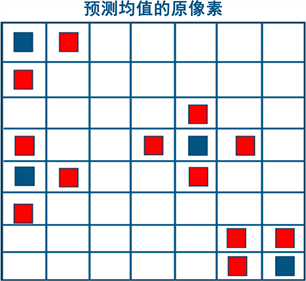Figure 1. Pixel prediction(a) 原始图像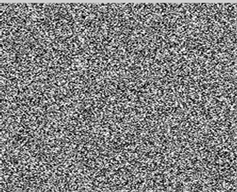(b) 加密图像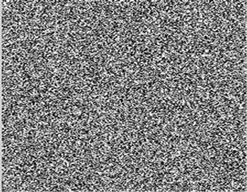(c) 嵌入数据的加密图像(d) 恢复图像

Figure 2. The simulation results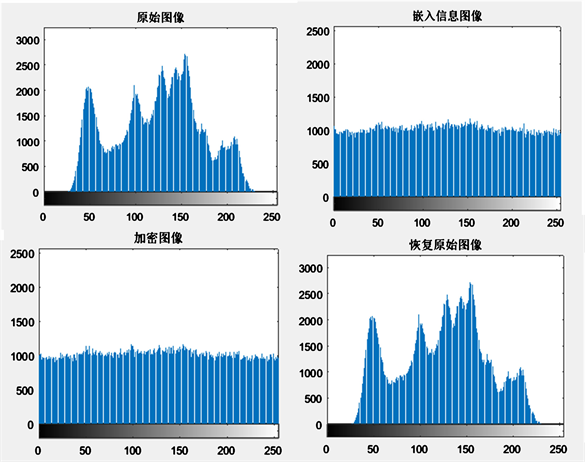Figure 3. Histogram results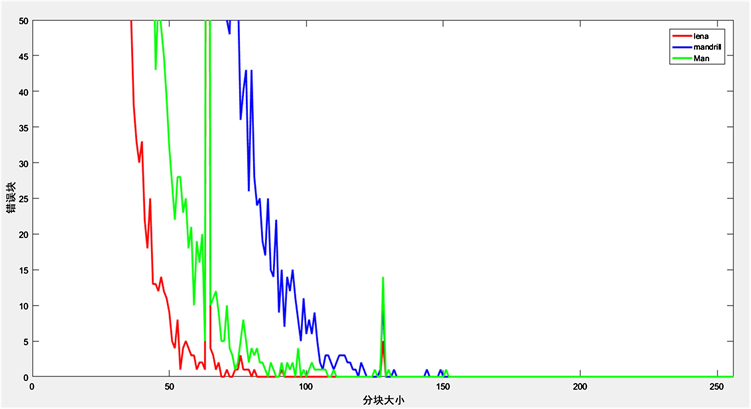Figure 4. Block size and information extraction error trend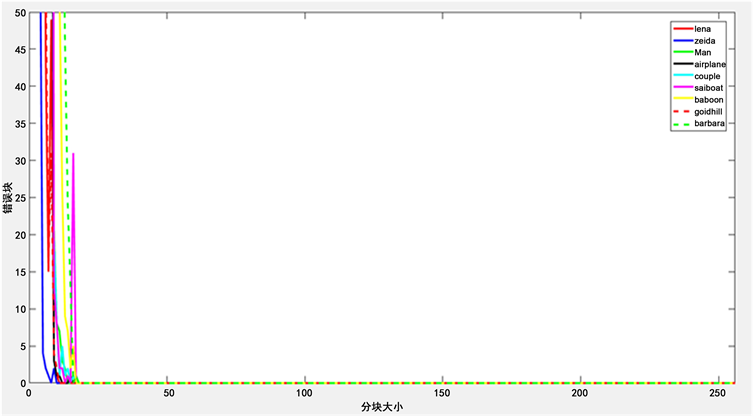Figure 5. Block size and information extraction error trend

4. 实验分析比较

$B\in \left(\frac{\left(x+1\right)A}{x}-\frac{255}{x},\frac{\left(x+1\right)A}{x}\right)$ 提取为0 (1)

$B\in \left(\frac{\left(x+1\right)A}{x},\frac{\left(x+1\right)A}{x}+\frac{255}{x}\right)$ 提取为1 (2)

5. 结论

The Mean-Based Ciphertext Domain Reversible Information Hiding Is Improved by Pixel Correlation[J]. 计算机科学与应用, 2018, 08(11): 1657-1664. https://doi.org/10.12677/CSA.2018.811182

1. 1. Zhang, W.M., Wang, H., Hou, D.D. and Yu, N.H. (2016) Reversible Data Hiding in Encrypted Images by Reversible Image Trans-formation. IEEE Transactions on Multimedia, 18, 1469-1479.

2. 2. Yi, S. and Zhou, Y. (2015) An Improved Reversible Data Hiding in Encrypted Images. IEEE China Summit and International Conference on Signal and Information Processing, Chengdu, 12-15 July 2015, 225-229. https://doi.org/10.1109/ChinaSIP.2015.7230396

3. 3. Qian, Z.X., Xu, H.S., Luo, X.Y. and Zhang, X.P. (2018) New Framework of Reversible Data Hiding in Encrypted JPEG Bitstreams. IEEE Transactions on Circuits & Systems for Video Technology, 99, 1-1. https://doi.org/10.1109/TCSVT.2018.2797897

4. 4. Agrawal, S. and Kumar, M. (2017) Mean Value Based Reversible Data Hid-ing in Encrypted Images. Optik, 130, 922-934. https://doi.org/10.1016/j.ijleo.2016.11.059

5. 5. Xiang, S.J. and Luo, X. (2017) Efficient Reversible Data Hiding in Encrypted Image with Public Key Cryptosystem. EURASIP Journal on Advances in Signal Pro-cessing, 2017, 59.

6. 6. Qian, Z.X. and Zhang, X.P. (2016) Reversible Data Hiding in Encrypted Images with Distributed Source En-coding. IEEE Transactions on Circuits and Systems for Video Technology, 26, 636-646. https://doi.org/10.1109/TCSVT.2015.2418611

7. 7. Qian, Z.X., Zhang, X.P. and Feng, G.R. (2016) Reversible Data Hiding in Encrypted Images Based on Progressive Recovery. IEEE Signal Processing Letters, 23, 1672-1676. https://doi.org/10.1109/LSP.2016.2585580

8. 8. Zhang, X.P., Qin, C. and Sun, G.L. (2012) Reversible Data Hiding in Encrypted Images Using Pseudorandom Sequence Modulation. Proceedings of IWDW, LNCS, Berlin, 358-367.

9. 9. Wu, X.T. and Sun, W. (2014) High-Capacity Reversible Data Hiding in Encrypted Images by Prediction Error. Signal Processing, 104, 387-400. https://doi.org/10.1016/j.sigpro.2014.04.032